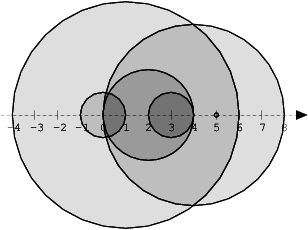# # codility

404개의 포스트## Lesson 6 - NumberOfDiscIntersections

We draw N discs on a plane. The discs are numbered from 0 to N − 1. An array A of N non-negative integers, specifying the radiuses of the discs, is gi

어제
·
0개의 댓글
·

## Lesson 6 - Triplet

An array A consisting of N integers is given. A triplet (P, Q, R) is triangular if 0 ≤ P &lt; Q &lt; R &lt; N and:AP + AQ > AR,AQ + AR > AP,AR + AP >

3일 전
·
0개의 댓글
·

A non-empty array A consisting of N integers is given.The leader of this array is the value that occurs in more than half of the elements of A.An equi

3일 전
·
0개의 댓글
·

## [Codility]Lesson5/PrefixSums/GenomicRangeQuery

https&#x3A;//app.codility.com/demo/results/trainingRNBDPW-72S/A DNA sequence can be represented as a string consisting of the letters A, C, G and T, w

3일 전
·
0개의 댓글
·

## [Codility]Lesson1/Iterations/BinaryGap

https&#x3A;//app.codility.com/demo/results/trainingV47U7M-RBU/A binary gap within a positive integer N is any maximal sequence of consecutive zeros th

3일 전
·
0개의 댓글
·

## [Codility]Lesson16/Greedy/MaxNonoverlappingSegments

Located on a line are N segments, numbered from 0 to N − 1, whose positions are given in arrays A and B. For each I (0 ≤ I &lt; N) the position of seg

4일 전
·
0개의 댓글
·

## [Codility]Lesson15/CaterpillorMethod/AbsDistinct

A non-empty array A consisting of N numbers is given. The array is sorted in non-decreasing order. The absolute distinct count of this array is the nu

4일 전
·
0개의 댓글
·

## [Codility]Lesson12/Euclidean algorithm/ChocolatesByNumbers

Two positive integers N and M are given. Integer N represents the number of chocolates arranged in a circle, numbered from 0 to N − 1.You start to eat

4일 전
·
0개의 댓글
·

## [Codility]Lesson11/SieveOfEratosthenes/CountNonDivisible

https&#x3A;//app.codility.com/demo/results/training9GEGBK-XZE/// you can also use imports, for example:// import java.util.\*;// you can write to stdo

5일 전
·
0개의 댓글
·

## [Codility]Lesson10/Prime and Composite Numbers/CountFactors

A positive integer D is a factor of a positive integer N if there exists an integer M such that N = D \* M.For example, 6 is a factor of 24, because M

5일 전
·
0개의 댓글
·

## Lesson 6 - MaxProductOfThree

A non-empty array A consisting of N integers is given. The product of triplet (P, Q, R) equates to AP AQ AR (0 ≤ P &lt; Q &lt; R &lt; N).For example

6일 전
·
0개의 댓글
·

## [Codility]Lesson9/MaximumSliceProblem

An array A consisting of N integers is given. It contains daily prices of a stock share for a period of N consecutive days. If a single share was boug

6일 전
·
0개의 댓글
·

## Lesson 6 - distinct

Write a functionclass Solution { public int solution(int\[] A); }that, given an array A consisting of N integers, returns the number of distinct value

6일 전
·
0개의 댓글
·

## Lesson 5 - MinAvgTwoSlice

A non-empty array A consisting of N integers is given. A pair of integers (P, Q), such that 0 ≤ P &lt; Q &lt; N, is called a slice of array A (notice

2023년 5월 23일
·
0개의 댓글
·

## Lesson 5 - GenomicRangeQuery

A DNA sequence can be represented as a string consisting of the letters A, C, G and T, which correspond to the types of successive nucleotides in the

2023년 5월 22일
·
0개의 댓글
·

## Lesson 5 - CountDiv

Write a function:class Solution { public int solution(int A, int B, int K); }that, given three integers A, B and K, returns the number of integers wit

2023년 5월 19일
·
0개의 댓글
·

## Lesson 5 - Prefix Sum

A non-empty array A consisting of N integers is given. The consecutive elements of array A represent consecutive cars on a road.Array A contains only

2023년 5월 17일
·
0개의 댓글
·

## Lesson 4 - MissingInteger

This is a demo task.Write a function:class Solution { public int solution(int\[] A); }that, given an array A of N integers, returns the smallest posit

2023년 5월 16일
·
0개의 댓글
·

## Lesson 4 - MaxCounters;

You are given N counters, initially set to 0, and you have two possible operations on them:increase(X) − counter X is increased by 1,max counter − all

2023년 5월 15일
·
0개의 댓글
·

## Lesson 4 - PermCheck

A non-empty array A consisting of N integers is given.A permutation is a sequence containing each element from 1 to N once, and only once.For example,

2023년 5월 15일
·
0개의 댓글
·